JAJSEU0G March   2003  – February 2018

PRODUCTION DATA.

1. 特長
2. アプリケーション
3. 概要
4. 改訂履歴
5. Pin Configuration and Functions
6. Specifications
7. Parameter Measurement Information
8. Detailed Description
1. 8.1 Overview
2. 8.2 Functional Block Diagram
3. 8.3 Feature Description
4. 8.4 Device Functional Modes
9. Application and Implementation
1. 9.1 Application Information
2. 9.2 Typical Application
10. 10Power Supply Recommendations
11. 11Layout
12. 12デバイスおよびドキュメントのサポート
1. 12.1 ドキュメントのサポート
2. 12.2 関連リンク
3. 12.3 コミュニティ・リソース
4. 12.4 商標
5. 12.5 静電気放電に関する注意事項
6. 12.6 Glossary
13. 13メカニカル、パッケージ、および注文情報

#### パッケージ・オプション

デバイスごとのパッケージ図は、PDF版データシートをご参照ください。

• D|8
• PW|8
• PW|8

### 6.13 Typical Characteristics

CONDITION STATEMENT TBD

### Table 1. Table of Graphs

GRAPH TITLE FIGURE
VIO Input offset voltage vs common-mode input voltage 1, 2
IIB Input bias current vs free-air temperature 3, 4
IIO Input offset current vs free-air temperature 3, 4
VOH High-level output voltage vs high-level output current 5, 6
VOL Low-level output voltage vs low-level output current 7, 8
VO(PP) Maximum peak-to-peak output voltage vs frequency 9, 10
Open-loop gain vs frequency 11, 12
Phase vs frequency 11, 12
AVD Differential voltage amplification vs load resistance 13
zo Output impedance vs frequency 15, 16
CMRR Common-mode rejection ratio vs frequency 17
kSVR Supply-voltage rejection ratio vs frequency 18, 19
IDD Supply current vs supply voltage 20
Supply current vs free-air temperature 21
Amplifier turnon characteristics 22
Amplifier turnoff characteristics 23
Supply current turnon 24
Supply current turnoff 25
Shutdown supply current vs free-air temperature 26
SR Slew rate vs load capacitance 27
Vn Equivalent input noise voltage vs frequency 28, 29
Equivalent input noise voltage vs common-mode input voltage 30, 31
THD Total harmonic distortion vs frequency 32, 33
THD + N Total harmonic distortion plus noise vs peak-to-peak signal amplitude 34, 35
φm Phase margin vs frequency 11, 12
Phase margin vs load capacitance 36
Phase margin vs free-air temperature 37
Gain-bandwidth product vs supply voltage 38
Gain-bandwidth product vs free-air temperature 39
Large signal follower 40, 41
Small signal follower 42, 43
Inverting large signal 44, 45
Inverting small signal 46, 47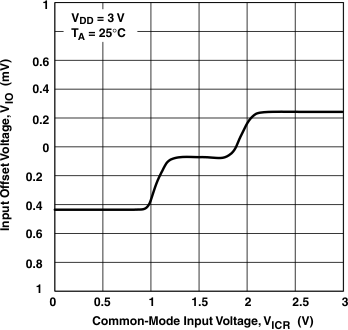VDD = 3 V
Figure 1. Input Offset Voltage vs Common-Mode Input Voltage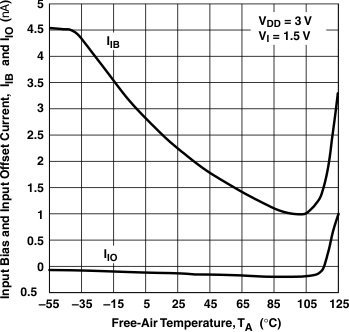VDD = 3 V
Figure 3. Input Bias and Input Offset Current vs Free-Air Temperature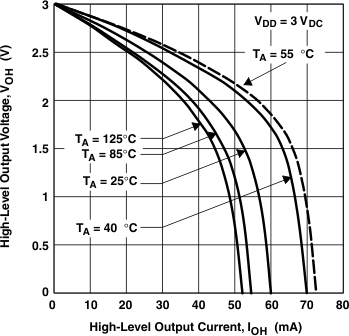VDD = 3 VDC
Figure 5. High-Level Output Voltage vs High-Level Output Current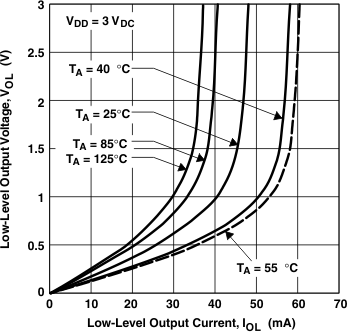VDD = 3 VDC
Figure 7. Low-Level Output Voltage vs Low-Level Output Current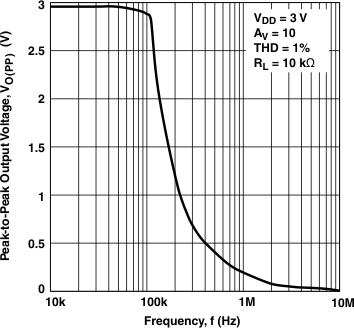VDD = 3 V
Figure 9. Peak-to-Peak Output Voltage vs Frequency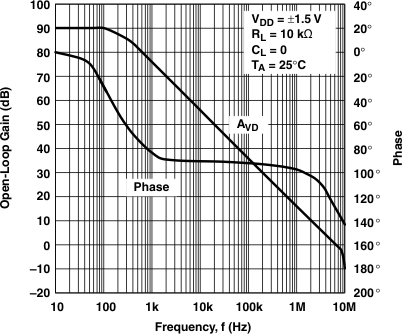VDD = ±1.5 V
Figure 11. Open-Loop Gain and Phase vs Frequency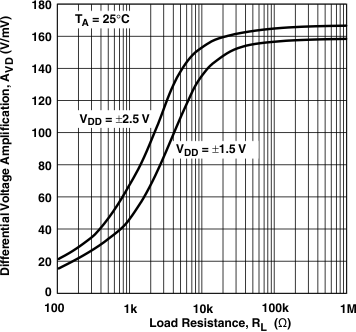Figure 13. Differential Voltage Amplification vs Load Resistance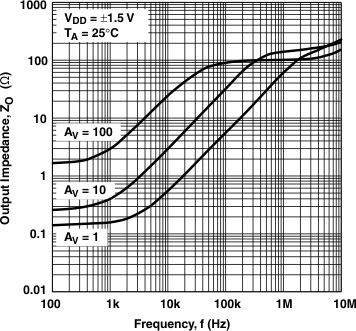VDD = ±1.5 V
Figure 15. Output Impedance vs Frequency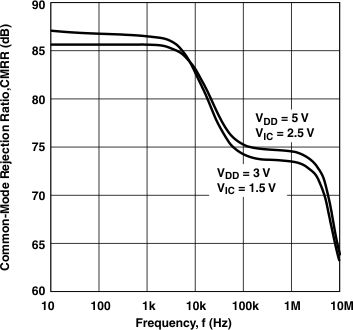Figure 17. Common-Mode Rejection Ratio vs Frequency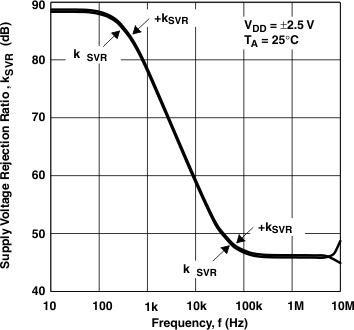VDD = ±2.5 V
Figure 19. Supply-Voltage Rejection Ratio vs Frequency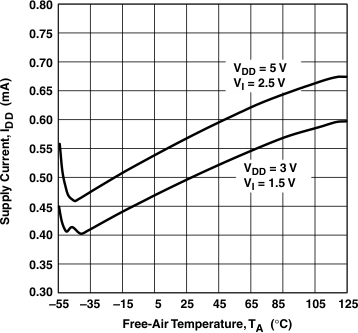Figure 21. Supply Current vs Free-Air Temperature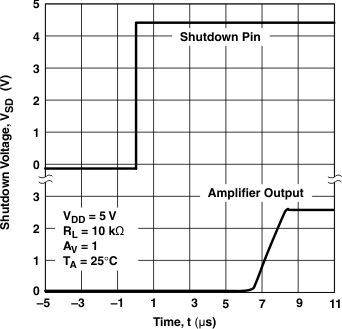Figure 23. Amplifier With a Shutdown Pulse Turnoff Characteristics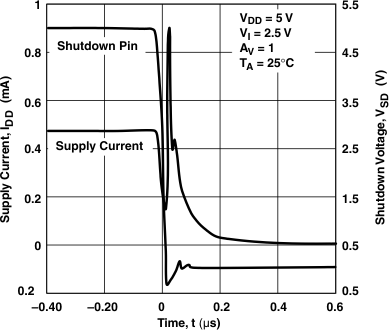Figure 25. Turnoff Supply Current With a Shutdown Pulse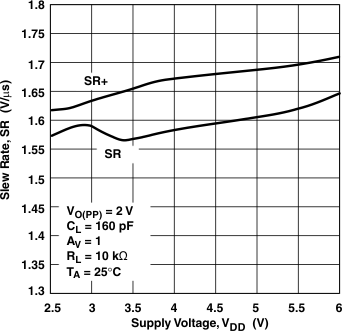Figure 27. Slew Rate vs Supply VoltageFigure 29. Equivalent Input Noise Voltage vs Frequency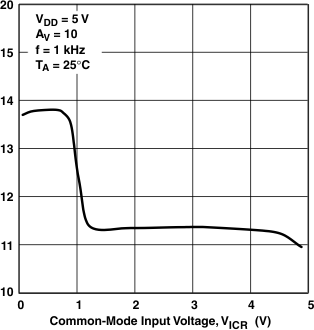Figure 31. Equivalent Input Noise Voltage vs Common-Mode Input Voltage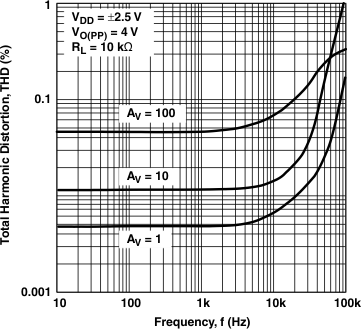VDD = ±2.5 V
Figure 33. Total Harmonic Distortion vs Frequency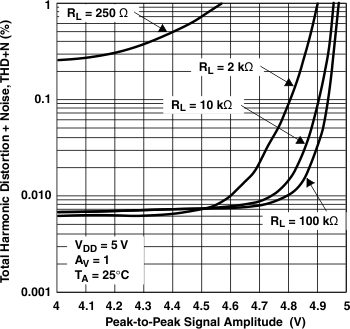VDD = 5 V
Figure 35. Total Harmonic Distortion Plus Noise vs Peak-to-Peak Signal AmplitudeFigure 37. Phase Margin vs Free-Air Temperature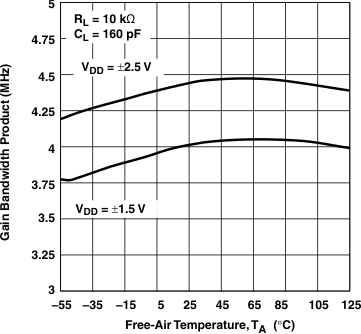Figure 39. Gain Bandwidth Product vs Free-Air Temperature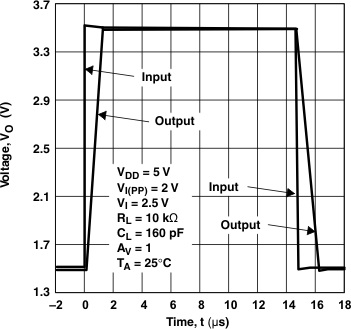VDD = 5 V
Figure 41. Large Signal Follower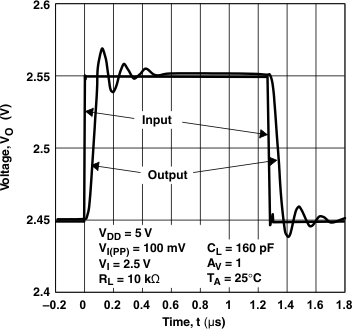VDD = 5 V
Figure 43. Small Signal Follower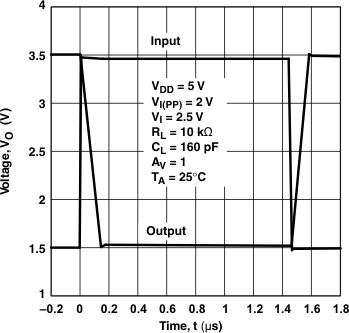VDD = 5 V
Figure 45. Inverting Large Signal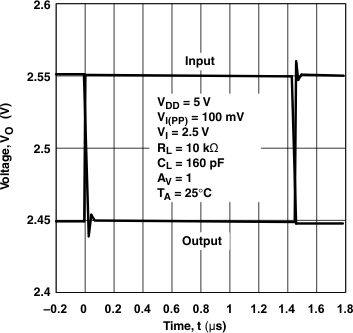VDD = 5 V
Figure 47. Inverting Small Signal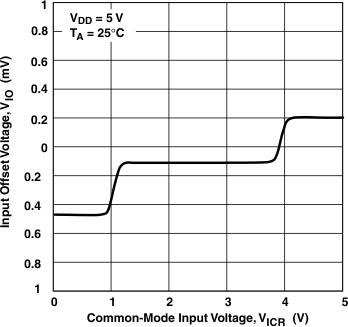VDD = 5 V
Figure 2. Input Offset Voltage vs Common-Mode Input VoltageVDD = 5 V
Figure 4. Input Bias and Input Offset Current vs Free-Air Temperature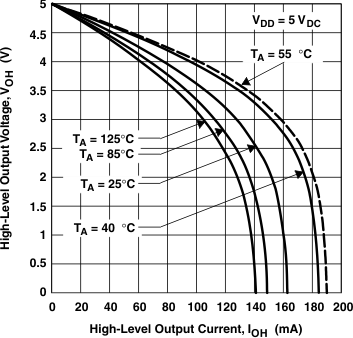VDD = 5 VDC
Figure 6. High-Level Output Voltage vs High-Level Output Current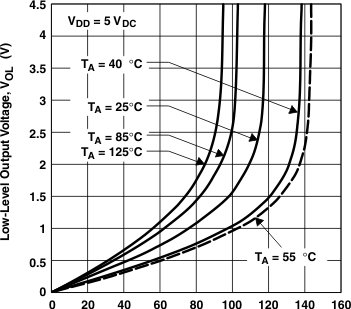VDD = 5 VDC
Figure 8. Low-level Output Voltage vs Low-Level Output CurrentVDD = 5 V
Figure 10. Peak-to-Peak Output Voltage vs Frequency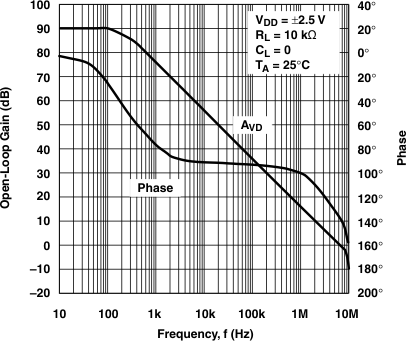VDD = ±2.5 V
Figure 12. Open-Loop Gain and Phase vs Frequency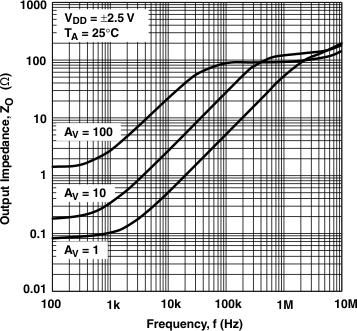VDD = ±2.5 V
Figure 16. Output Impedance vs FrequencyVDD = ±1.5 V
Figure 18. Supply-Voltage Rejection Ratio vs Frequency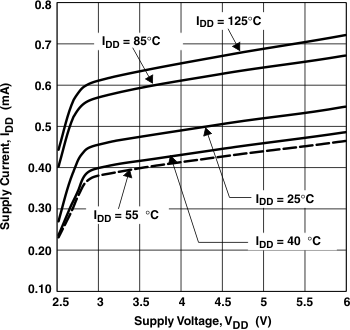Figure 20. Supply Current vs Supply VoltageFigure 22. Amplifier With a Shutdown Pulse Turnon Characteristics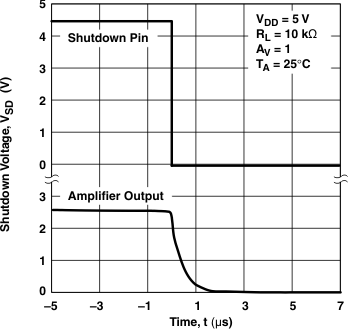Figure 24. Supply Current With a Shutdown Pulse Turnon Characteristics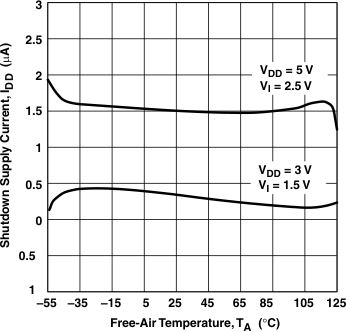Figure 26. Shutdown Supply Current vs Free-Air Temperature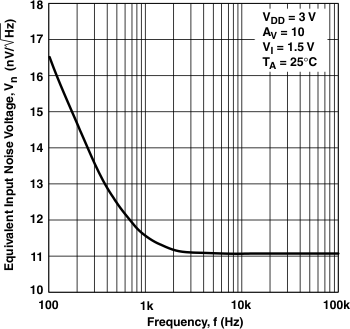Figure 28. Equivalent Input Noise Voltage vs Frequency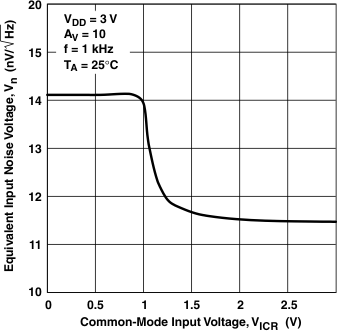Figure 30. Equivalent Input Noise Voltage vs Common-Mode Input Voltage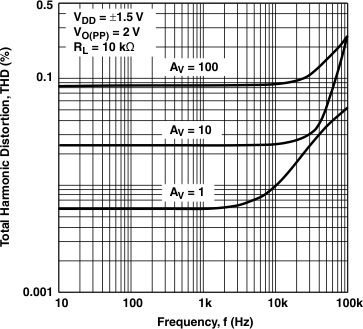VDD = ±1.5 V
Figure 32. Total Harmonic Distortion vs FrequencyVDD = 3 V
Figure 34. Total Harmonic Distortion Plus Noise vs Peak-to-Peak Signal Amplitude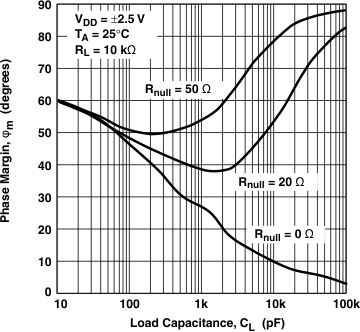Figure 36. Phase Margin vs Load Capacitance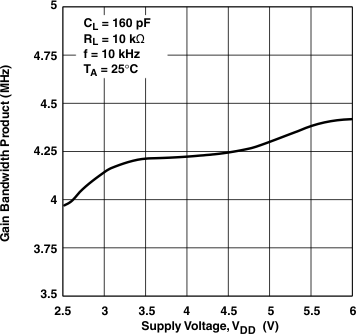Figure 38. Gain Bandwidth Product vs Supply Voltage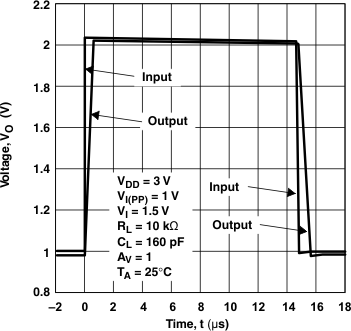VDD = 3 V
Figure 40. Large Signal Follower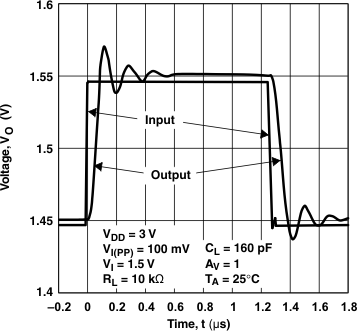VDD = 3 V
Figure 42. Small Signal Follower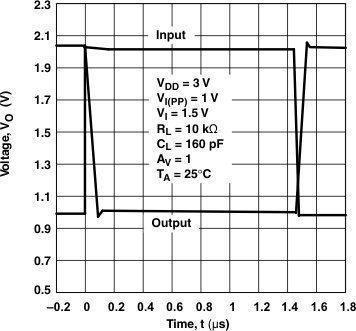VDD = 3 V
Figure 44. Inverting Large Signal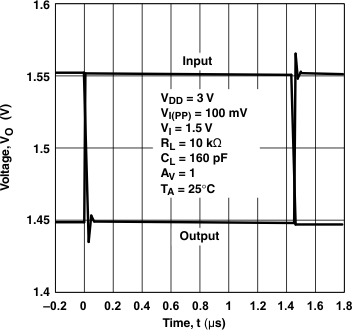VDD = 3 V
Figure 46. Inverting Small Signal